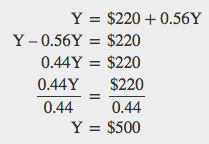## Equilibrium in the Keynesian Cross Model

With the aggregate expenditure line in place, the next step is to relate it to the two other elements of the Keynesian cross diagram. Thus, the first subsection interprets the intersection of the aggregate expenditure function and the 45-degree line, while the next subsection relates this point of intersection to the potential GDP line.

### WHERE EQUILIBRIUM OCCURS

The point where the aggregate expenditure line that is constructed from C + I + G + X – M crosses the 45-degree line will be the equilibrium for the economy. It is the only point on the aggregate expenditure line where the total amount being spent on aggregate demand equals the total level of production. In Figure B.8, this point of equilibrium (E0) happens at 6,000, which can also be read off Table B.3.

The meaning of “equilibrium” remains the same; that is, equilibrium is a point of balance where no incentive exists to shift away from that outcome. To understand why the point of intersection between the aggregate expenditure function and the 45-degree line is a macroeconomic equilibrium, consider what would happen if an economy found itself to the right of the equilibrium point E, say point H in Figure B.8, where output is higher than the equilibrium. At point H, the level of aggregate expenditure is below the 45-degree line, so that the level of aggregate expenditure in the economy is less than the level of output. As a result, at point H, output is piling up unsold—not a sustainable state of affairs.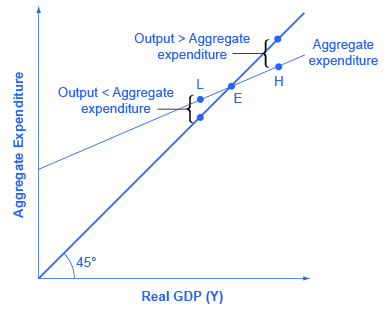Figure B.8. Equilibrium in the Keynesian Cross Diagram. If output was above the equilibrium level, at H, then the real output is greater than the aggregate expenditure in the economy. This pattern cannot hold, because it would mean that goods are produced but piling up unsold. If output was below the equilibrium level at L, then aggregate expenditure would be greater than output. This pattern cannot hold either, because it would mean that spending exceeds the number of goods being produced. Only point E can be at equilibrium, where output, or national income and aggregate expenditure, are equal. The equilibrium (E) must lie on the 45-degree line, which is the set of points where national income and aggregate expenditure are equal.

Conversely, consider the situation where the level of output is at point L—where real output is lower than the equilibrium. In that case, the level of aggregate demand in the economy is above the 45-degree line, indicating that the level of aggregate expenditure in the economy is greater than the level of output. When the level of aggregate demand has emptied the store shelves, it cannot be sustained, either. Firms will respond by increasing their level of production. Thus, the equilibrium must be the point where the amount produced and the amount spent are in balance, at the intersection of the aggregate expenditure function and the 45-degree line.

### FINDING EQUILIBRIUM

The following gives some information on an economy. The Keynesian model assumes that there is some level of consumption even without income. That amount is $236 –$216 = $20.$20 will be consumed when national income equals zero. Assume that taxes are 0.2 of real GDP. Let the marginal propensity to save of after-tax income be 0.1. The level of investment is $70, the level of government spending is$80, and the level of exports is $50. Imports are 0.2 of after-tax income. Given these values, you need to complete the table and then answer these questions: What is the consumption function? What is the equilibrium? Why is a national income of$300 not at equilibrium? How do expenditures and output compare at this point?

National Income Taxes After-tax income Consumption I + G + X Imports Aggregate Expenditures
$300$236
$400$500
$600$700

Step 1. Calculate the amount of taxes for each level of national income(reminder: GDP = national income) for each level of national income using the following as an example: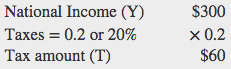Step 2. Calculate after-tax income by subtracting the tax amount from national income for each level of national income using the following as an example: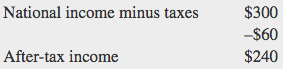Step 3. Calculate consumption. The marginal propensity to save is given as 0.1. This means that the marginal propensity to consume is 0.9, since MPS + MPC = 1. Therefore, multiply 0.9 by the after-tax income amount using the following as an example: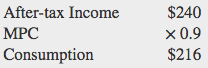Step 4. Consider why the table shows consumption of $236 in the first row. As mentioned earlier, the Keynesian model assumes that there is some level of consumption even without income. That amount is$236 – $216 =$20.

Step 5. There is now enough information to write the consumption function. The consumption function is found by figuring out the level of consumption that will happen when income is zero. Remember that:

C = Consumption when national income is zero + MPC (after-tax income)

Let C represent the consumption function, Y represent national income, and T represent taxes.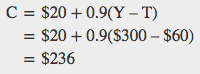Step 6. Use the consumption function to find consumption at each level of national income.

Step 7. Add investment (I), government spending (G), and exports (X). Remember that these do not change as national income changes:

Step 8. Find imports, which are 0.2 of after-tax income at each level of national income. For example: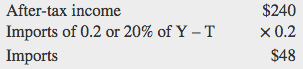Step 9. Find aggregate expenditure by adding C + I + G + X – I for each level of national income. Your completed table should look like this:

National Income (Y) Tax = 0.2 × Y (T) After-tax income (Y – T) Consumption C = $20 + 0.9(Y – T) I + G + X Minus Imports (M) Aggregate Expenditures AE = C + I + G + X – M$300 $60$240 $236$200 $48$388
$400$80 $320$308 $200$64 $444$500 $100$400 $380$200 $80$500
$600$120 $480$452 $200$96 $556$700 $140$560 $524$200 $112$612

Step 10. Answer the question: What is equilibrium? Equilibrium occurs where AE = Y. This table shows that equilibrium occurs where national income equals aggregate expenditure at $500. Step 11. Find equilibrium mathematically, knowing that national income is equal to aggregate expenditure.Step 10. Answer the question: What is equilibrium? Equilibrium occurs where AE = Y. The table shows that equilibrium occurs where national income equals aggregate expenditure at$500.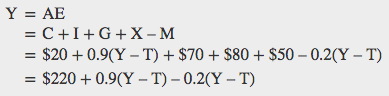Since T is 0.2 of national income, substitute T with 0.2 Y so that: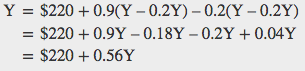Solve for Y.